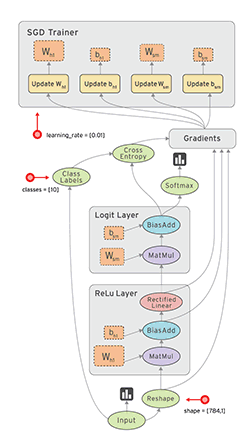# Curiousily

## TensorFlow Basics | TensorFlow for Hackers (Part I)

Deep Learning, Neural Networks, TensorFlow, Python3 min read

Share

The ONLY way to understand TensorFlow is by watching the following video:

Now, I can safely assume that we are at the same level of understanding. Ready to fire up some tensors?

# .css-kfjr0z{color:var(--theme-ui-colors-primary,#3182ce);-webkit-text-decoration:none;text-decoration:none;}.css-kfjr0z:hover{-webkit-text-decoration:underline;text-decoration:underline;}.css-kfjr0z svg{fill:var(--theme-ui-colors-secondary,#4a5568);margin-right:0.5rem;}What is TensorFlow?

TensorFlow is a library for number crunching created and maintained by Google. It’s used mainly for machine learning (especially deep learning) tasks. While still in beta (version 1.0 is currently in alpha), the library was open sourced more than a year ago (November 9, 2015). Since then it pretty much took the Deep Learning (DL) community by a storm. Tons of companies are using it in production, also. The best place to learn more is the official page of TensorFlow.

On the more technical side, TensorFlow allows you to do computations on your PC/Mac (CPU & GPU), Android, iOS and lots more places. Of course, being created by Google, it aims to bring massive parallelism to your backprop musings. The main abstraction behind all the magic is stateful dataflow graphs.Your data flowing through a graph in TensorFlow

## Eh, Tensors?

The glossary of TensorFlow states that a tensor is:

A Tensor is a typed multi-dimensional array. For example, a 4-D array of floating point numbers representing a mini-batch of images with dimensions [batch, height, width, channel].

So, you can think of a tensor as a matrix on steroids - expanded to $n$ more dimensions. The concept might feel a bit strange at first, but don’t worry it will come around eventually.

# Installing TensorFlow

If you want basic installation without all the fuss, just do this:

1pip install tensorflow

Or install it with GPU support:

1pip install tensorflow-gpu

Otherwise, you might have a look here if you want to build from source (might need this if you want to support custom cuDNN version) or whatever.

Now that you have everything installed. Let’s check that we can import TensorFlow.

1import tensorflow as tf2import numpy as np3import matplotlib.pyplot as plt4%matplotlib inline
1tf.__version__
1'1.0.0-alpha'

Success! As you can see, I am using version 1.0 alpha. Let’s get those tensors flowing.

# Bringing the big guns

Writing TensorFlow code might require some getting use to at first. There are some concepts that you must familiarize yourself with.

### Variables

Variables are pretty standard stuff. You just have to remember one thing - define them before using them in the computational graph.

### Placeholders

Placeholders are used to feed in data from outside the computational graph. So, if you need to pass data to the model from outside TensorFlow, you have to define a placeholder. Each placeholder must specify a data type. You specify your data using feed_dict when running your computation.

### Session

In order to run any meaningful operation on your graph, you need a Session. In sessions, we trust (not cookies), most of the time. Here is a short example:

1v1 = tf.Variable(0.0)2p1 = tf.placeholder(tf.float32)3new_val = tf.add(v1, c1)4update = tf.assign(v1, new_val)56with tf.Session() as sess:7    sess.run(tf.global_variables_initializer())8    for _ in range(5):9        sess.run(update, feed_dict={p1: 1.0})10    print(sess.run(v1))
15.0

# Simple Linear Regression in TensorFlow

This very well known model is a good way to start your exploration in TensorFlow. It is described by the following equation:

$Y = aX + b$

Where Y is the dependent and X is the independent variable. Our task is to adjust the parameters a - “slope” and b - “intercept” so that we best describe the data using a line.

For our example, let’s find out how eating burgers affect your resting heart rate. The data will be simulated, so no conclusions, please! Our data represents the average number of burgers eaten per day.

1X = np.random.rand(100).astype(np.float32)

The slope and intercept we are looking for are respectively $a = 50$ and $b = 40$.

1a = 502b = 403Y = a * X + b

Let’s have a look at what our model should figure out:

1plt.plot(X, Y);

Let’s make things a tiny bit more interesting by adding a bit of noise to our dependent variable.

1Y = np.vectorize(lambda y: y + np.random.normal(loc=0.0, scale=0.05))(Y)
1a_var = tf.Variable(1.0)2b_var = tf.Variable(1.0)3y_var = a_var * X + b_var

Our task will be to minimize the mean squared error or in TensorFlow parlance - reduce the mean.

1loss = tf.reduce_mean(tf.square(y_var - Y))

So, let’s try to minimize it using gradient descent.

1optimizer = tf.train.GradientDescentOptimizer(0.5)2train = optimizer.minimize(loss)

Let’s use our optimizer for 300 steps of learning

1TRAINING_STEPS = 3002results = []3with tf.Session() as sess:4    sess.run(tf.global_variables_initializer())5    for step in range(TRAINING_STEPS):6        results.append(sess.run([train, a_var, b_var])[1:])

Let’s get the final and best predictions for $a$ and $b$

1final_pred = results[-1]2a_hat = final_pred3b_hat = final_pred4y_hat = a_hat * X + b_hat56print("a:", a_hat, "b:", b_hat)
1a: 50.0 b: 40.0
1plt.plot(X, Y);2plt.plot(X, y_hat);